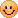## TCO11 Qual1

TopCoder Open 2011 시작!!
작년에 TCO10 때 휴가까지 쓰고 참가했는데도 불구하고 Qual round 도 통과못한 아쉬움이 있었는데..
올해도 상황을 보니 작년과 비슷하게 진행되고 있다.. -_-
맞았다고 생각한 500 이 의외로 fail 하면서 637 등.. 간발의 차이로 탈락했다.. 젠장!!!!
500 이 fail 한 이유는 특정 케이스에 대해서 TLE 가 나는거였는데.. 나와 같은 이유로 fail 한 사람이 많았다..
이런 xx 같은 경우가 다 있다니..!!Level1 - MinimumLiars

i번째 사람이 liar 라면 claim[i] 는 전체 liar 수보다 크고, i번째 사람이 liar 가 아니라면 claim[i] 는 전체 liar 수보다 작거나 같다.. 최소 가능한 liar 수 구하기

liar 수를 0 ~ n 까지 넣고 시도해보았다..

1 #include <iostream>
2 #include <cstdio>
3 #include <algorithm>
4 #include <vector>
5 #include <string>
6 using namespace std;
7 //#define min(x, y) ((x) > (y) ? (y) : (x))
8 //#define max(x, y) ((x) > (y) ? (x) : (y))
9 //#define INF 999999999
10 //#define EPS 1e-14
11
12 class MinimumLiars {
13 public:
14
15 int getMinimum(vector <int> claim)
16 {
17     int n;
18     int i, j;
19     int cnt;
20     n = claim.size();
21     for (i = 0; i <= n; i++) {
22         cnt = 0;
23         for (j = 0; j < n; j++) {
24             if (claim[j] <= i) {
25             }
26             else {
27                 cnt++;
28             }
29         }
30         if (cnt == i) {
31             return i;
32         }
33     }
34     return -1;
35 }
36
37 };

Level2 - FoxListeningToMusic

각 음악의 재생 길이가 나오고.. random play 를 할때, T+0.5 초 순간에 i 번째 음악이 play 될 확률 구하기..

전형적인 DP 로 풀었다..
P[i][j] = i 초에 j 음악이 play 될 확률이라고 놓고 풀었다..

최악의 경우 loop 가 2억번 돌아서 약간 불안했는데.. tc 를 50개 다 넣어봐도 잘 돌아가길래 submit
근데 특정 case 에서 fail 했다.. ㅠ_ㅠ;
double 값이 너무 작아지면 연산 속도가 갑자기 떨어지는 것 같다.. ㅠ_ㅠ;; (링크
그래서 너무 작은값이 나올때 무시하고 돌렸다..
문제 출제자.. 이건 좀 너무하잖아!!

1 #include <iostream>
2 #include <cstdio>
3 #include <algorithm>
4 #include <vector>
5 #include <string>
6 using namespace std;
7 #define min(x, y) ((x) > (y) ? (y) : (x))
8 //#define max(x, y) ((x) > (y) ? (x) : (y))
9 //#define INF 999999999
10 //#define EPS 1e-14
11
12 int n;
13 int tt;
14 double P;
15
16 class FoxListeningToMusic {
17 public:
18
19 vector <double> getProbabilities(vector <int> length, int T)
20 {
21     int i, j, k;
22     int temp;
23     double p;
24     vector<double> res;
25     n = length.size();
26     tt = T;
27     p = 1.0 / (double)n;
28     for (i = 0; i < n; i++) {
29         res.push_back(0);
30     }
31     for (i = 0; i <= T; i++) {
32         for (j = 0; j < n; j++) {
33             P[i][j] = 0.0;
34         }
35     }
36     for (i = 0; i < n; i++) {
37         if (length[i] > T)
38             res[i] += p;
39         else
40             P[length[i]][i] = p;
41     }
42     for (i = 1; i <= T; i++) {
43         for (j = 0; j < n; j++) {
44             temp = i+length[j];
45             for (k = 0; k < n; k++) {
46                 if (P[i][k] > 1e-15) {
47                     if (temp > T)
48                         res[j] += P[i][k] * p;
49                     else
50                         P[temp][j] += P[i][k] * p;
51                 }
52             }
53         }
54     }
55     return res;
56 }
57
58 };

Level3 - SquareSeries

to be updated..

#### 'Problem Solving > TopCoder logs' 카테고리의 다른 글

 SRM 511  (0) 2011.07.04 2011.06.23 2011.06.09 2011.05.30 2011.05.20 2011.05.15 2011.04.27 2011.04.18 2011.04.01 2011.02.27 2011.02.02

1. kureyo 2011.05.16 17:24

안녕하세요, 오랜만이에요사실 med같은 경우는 O(TNN)이 아니라 O(TN)솔루션이 있어서 그걸로 풀라는게 아니었을지;;
저는 반대로 TNN에 T=80000,N=50으로 챌린지 시도하다가 실패했네요. ㅠㅠ
TCO서버가 애매하게 좋아져서(?) 특수한 데이타에서 걸리나봐요.

• 2011.05.16 23:32 신고

앗.. 1라운드 진출 축하합니다..~ 오프라인매치까지 고고..~